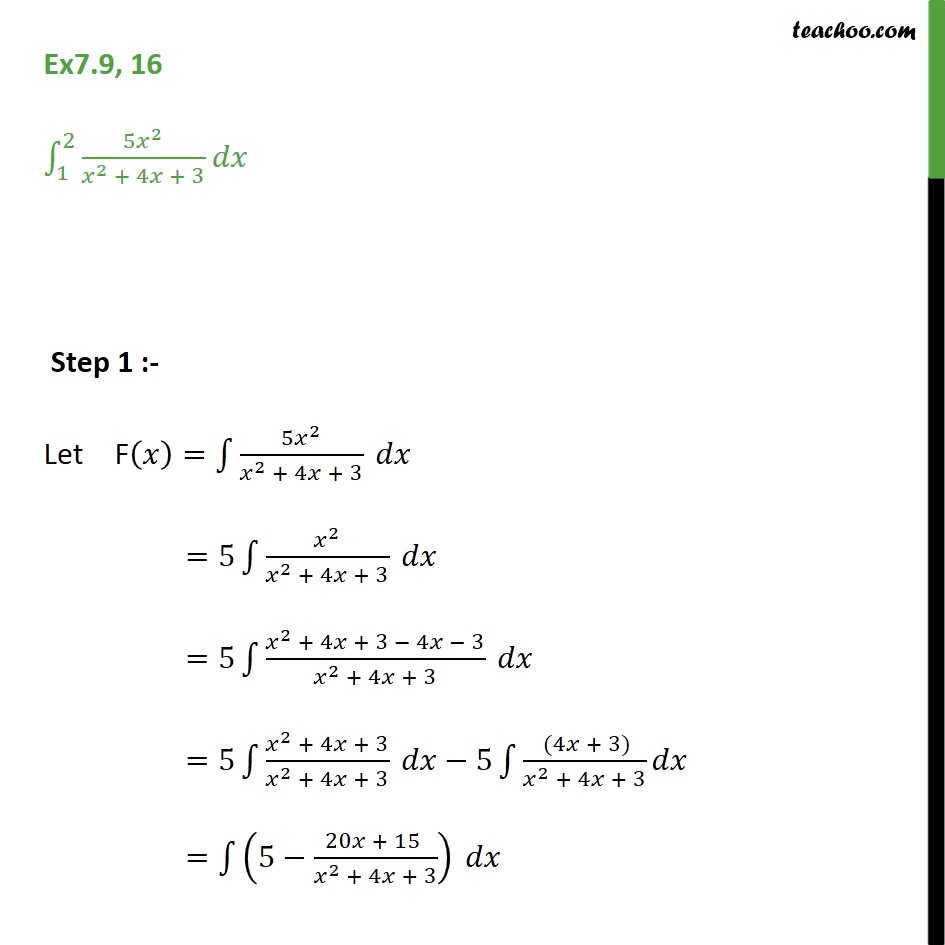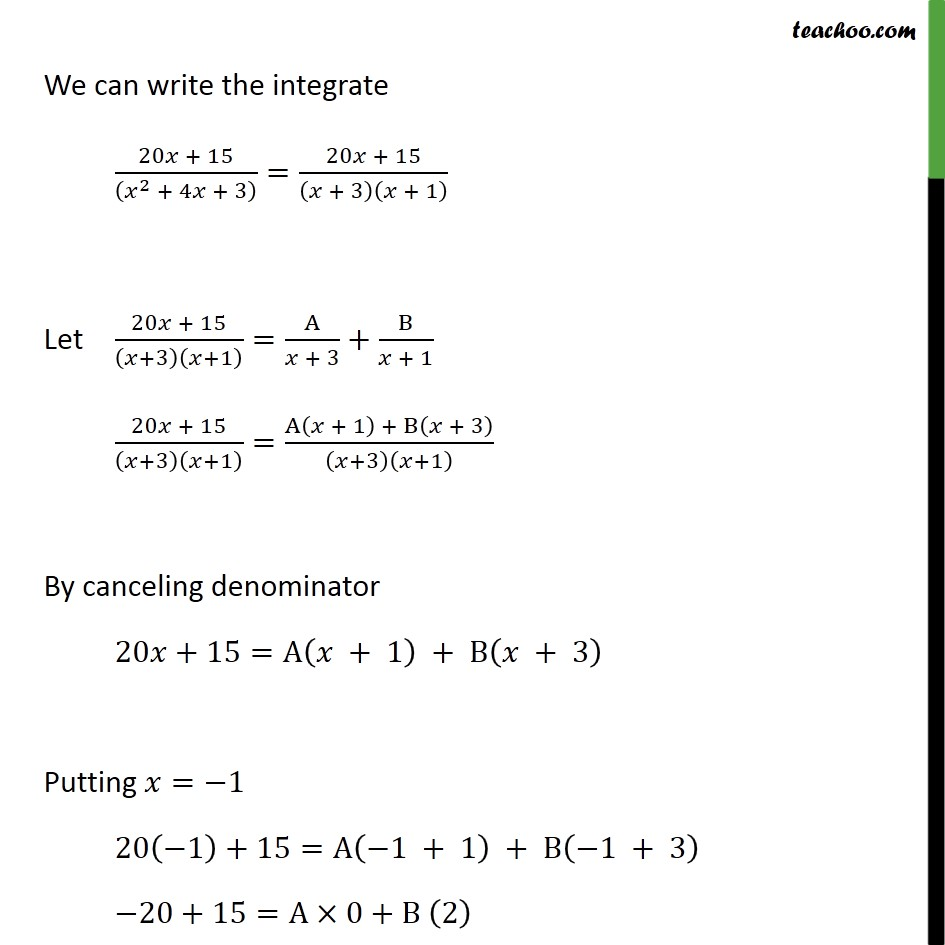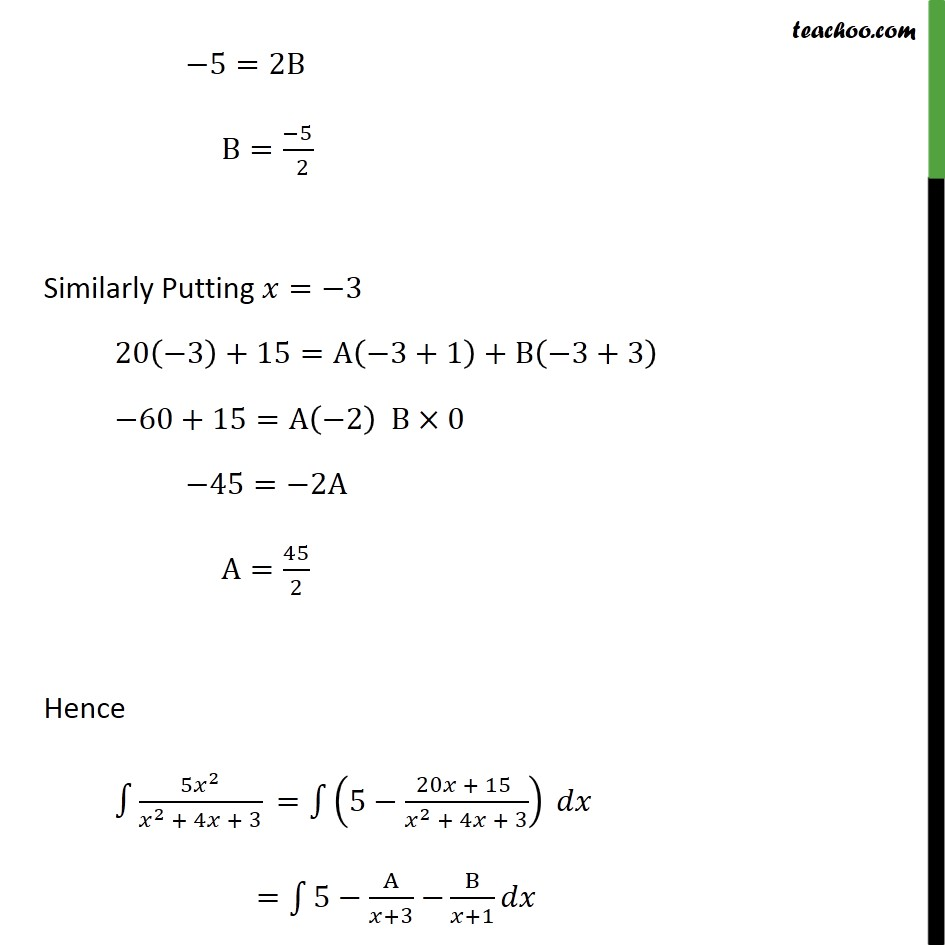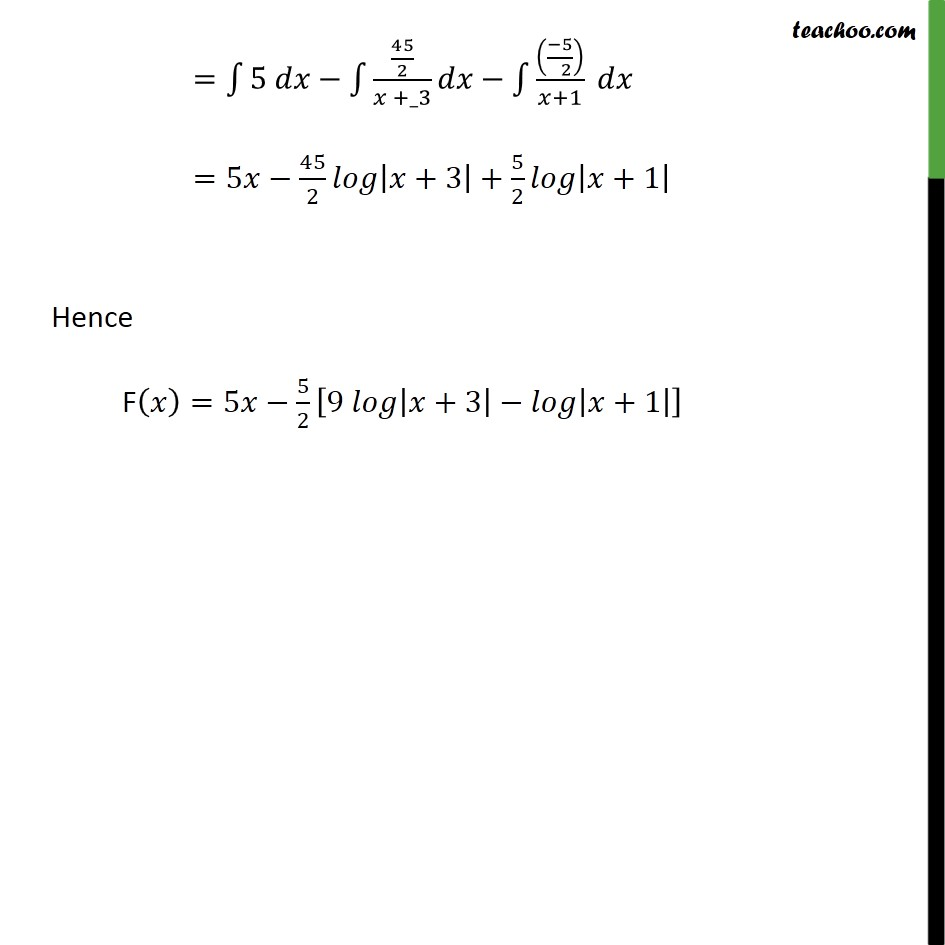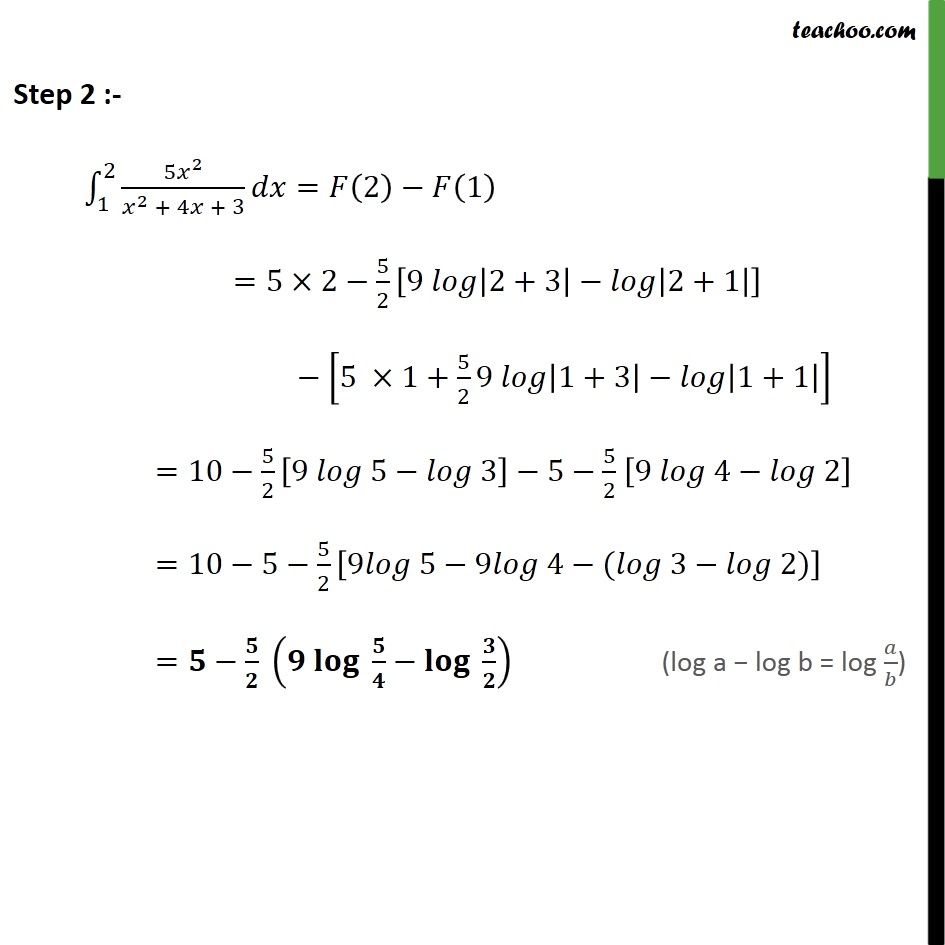1. Chapter 7 Class 12 Integrals
2. Serial order wise
3. Ex 7.9

Transcript

Ex7.9, 16 1 2 5 2 2 + 4 + 3 Step 1 :- Let F = 5 2 2 + 4 + 3 =5 2 2 + 4 + 3 =5 2 + 4 + 3 4 3 2 + 4 + 3 =5 2 + 4 + 3 2 + 4 + 3 5 (4 + 3) 2 + 4 + 3 = 5 20 + 15 2 + 4 + 3 We can write the integrate 20 + 15 2 + 4 + 3 = 20 + 15 + 3 + 1 Let 20 + 15 +3 +1 = A + 3 + B + 1 20 + 15 +3 +1 = A + 1 + B + 3 +3 +1 By canceling denominator 20 +15=A + 1 + B + 3 Putting = 1 20 1 +15=A 1 + 1 + B 1 + 3 20+15=A 0+B 2 5=2B B= 5 2 Similarly Putting = 3 20 3 +15=A 3+1 +B 3+3 60+15=A 2 B 0 45= 2A A= 45 2 Hence 5 2 2 + 4 + 3 = 5 20 + 15 2 + 4 + 3 = 5 A +3 B +1 = 5 45 2 +_3 5 2 +1 =5 45 2 +3 + 5 2 +1 Hence F =5 5 2 9 +3 +1 Step 2 :- 1 2 5 2 2 + 4 + 3 = 2 1 =5 2 5 2 9 2+3 2+1 5 1+ 5 2 9 1+3 1+1 =10 5 2 9 5 3 5 5 2 9 4 2 =10 5 5 2 9 5 9 4 ( 3 2) =

Ex 7.9

Chapter 7 Class 12 Integrals
Serial order wise